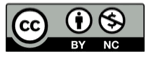# 外摆线的动态演示

2018-06-17## 参数表达式

$$x(t)=(R+r) \cos (t) - r \cos ({{R+r} \over r}t)\\y(t)=(R+r) \sin (t) - r \sin ({{R+r} \over r}t)$$

## 精彩评论